Please Note: Blog posts are not selected, edited or screened by Seeking Alpha editors.

# Hedging Options In A Trinomial Pricing Model (Pt. 1)

Summary

We all know it is possible to hedge Options on a binomial model, but can it be done on a trinomial model?

How does a Curved Quadratic Payoff become integrated in Linearity Principle?

How do we linearize the quadratic payoff without resorting to passing to the continuous time limit?

Preface

The introduction of the Rendleman and Bartter's binomial pricing model in 1979 and Cox, Ross and Rubinstein's a year later, gave new insight into the world of theoretical option pricing. Upon reading the proofs for these models and variations, the possibility of hedging an option is generally shown to be possible alongside the presentation of a 1 to 2 period binomial tree. The presentation of such a subject is unfortunately not given when considering a Trinomial model. This sparks the question, is it possible to hedge options in a Trinomial model?

Considerations

The use of Trinomial models are popularized due to their ability to converge faster than binomial models. Essentially they take a shorter time to achieve a given accuracy. The extra "branch" gives the model more freedom as well as the possibility to place nodes on barriers. So far the solution to our problem seems relatively simple since Trinomial models share several of the same properties as binomial models. So shouldn't hedging be the same? Not quite since we must not forget that the trader cannot hedge options using dynamic trading in just a stock and a bond. So is there a possible way to price?

Three Assets?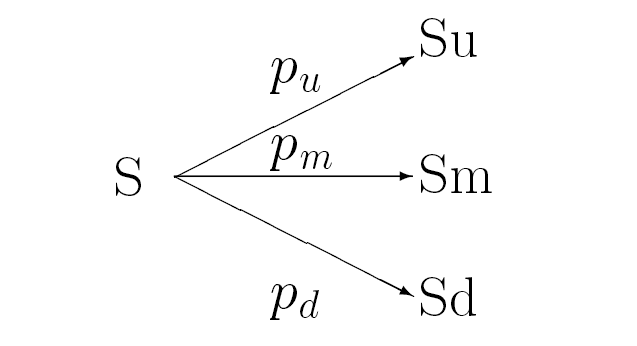In the common binomial model, two assets are used to hedge a derivative when there are two states. Consequently, when three states are present, a derivative can be hedged by using the corresponding amount of assets, in this case three.

This process can be easily observed with a single period expiration setting. Assume that interest rates are zero and that a stock price can either double, halve or remain the same at a price of \$2. In order to hedge a call with a sticking price of 3 we must consider using a bond, a stock and an "at the money" call with an intrinsic value of 2/3.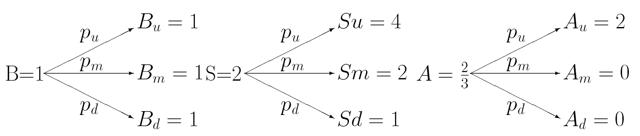Let's denote "Nb" as the number of bonds, "Ns" as the number of shares and "Na" as the number of at-the- money calls held in the hedge portfolio. If we equate the portfolio values to the desired payoffs in the three instances we are lefts with three linear equations with respect to the three unknowns.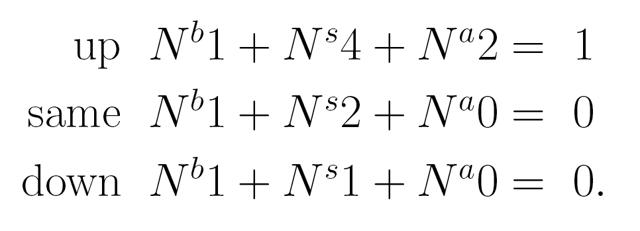The solution to this system of equations is "Nb = 0", "Ns = 0" and "Na = 1/2". Therefore given the initial price of the at-the-money call is \$2/3, we consider the arbitrage-free value of the call struck at \$3 to be \$1/3.

Two Periods Can be Trouble

Let's consider the re-combining, two period trinomial tree as shown below:

To keep matters simple, let's assume that the gross periodic stock returns the following: up "u>1", same "m=1" and down "d=1/u". Let's also assume that these variables are constant over time and that interest rates and dividend yields are zero.

If we follow the backward recursion used in the binomial model, we quickly encounter a problem. If we place ourselves at the upper node and the middle date, only 3 possible prices are presented. At a first glance, the situation appears to be identical to the single period problem, however, there is a subtle difference when using the value of a call. That is to say, in the single period problem, the price of the "at-the-money" call was known and consequently used to derive the value of a call with another strike. In the two period model, the time 1 price of any option is unknown due to the fact that known prices are found explicitly at the "root" of our trinomial tree. At the aforementioned middle date upper node, there are three possible future stock prices but only two known time 1 prices.

Integration of Linearity Principle

The way out of this mess is to pull out the "secret weapon" of derivative pricing; Linearity Principle. If we imagine a two period binomial model with zero interest rates and dividends, the principle thus states that if the derivative's payoff at the end of the second period is linear in the stock price, then the beginning value must be linear in the initial stock price to avoid arbitrage. That is to say:

If: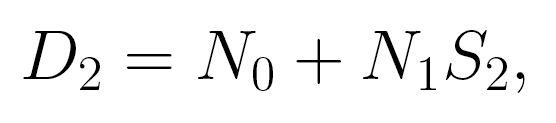Then:Although this is useful, we must now ask ourselves how can the principle be used to price a derivative with a nonlinear payoff.

Butterfly Spreads & Quadratic Payoffs

Let's review a quick example before tackling the problem. Assuming that a derivative's payoff at the end of the second period is the quadratic in the stock price below: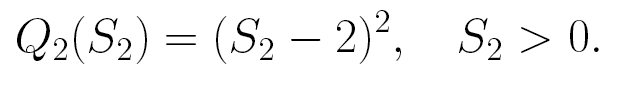If we were to theoretically graph this equation showing 5 possible payouts of a given stock price that starts at \$2 and can either double, halve or stay the same, we would observe an upward approaching exponential curve where the payoff is simply the stock price squared and subtracted by 2. The more the proportions increase the larger the gap between points on the graph. This makes taking the derivative or finding a specific limit of a point inefficient leading to the introduction of butterfly spreads.

Basically there are two methods that can be used to linearize the curved quadratic payout without resorting to passing the continuous time limit. (Which is complicated enough as it is.) This article will be focused solely on the steps of the first method since the second involves added introduction of topics. Both methods, however, are made easier to understand by applying the notion of a butterfly spread whose payoff is shown in the graph below: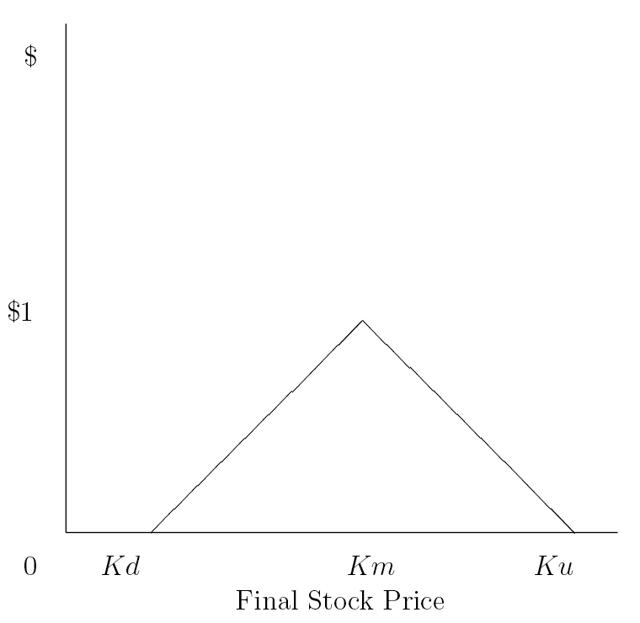Since the "zeros" or endpoint of the spread can be placed at any option strike price and the height of the vertex at any arbitrary point, we will consider butterfly spreads with endpoints at possible stock prices and heights of one.

Linearizing with Butterfly Spreads

In the first method of linearization of the curved quadratic payoff we will draw a line through any 2 of the 5 possible payoffs in a theoretical graph and then use static positions in the butterfly spreads centered at the remaining 3 to draw the payoff line. (Remember the quadratic payoff resembles an exponential function approaching positive infinity.)

Let's jump right into the equations, assume "N0" and "N1" to be the respective intercept and slope of the linearized payoff.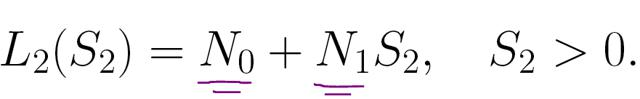As an example, if the second and third points from the left on our graph are used to draw a line, then the theoretical line should go through ( "Sdm" , "Ddm") = (1,1) and ("Smm" , "Dmm") = (2,0). This gives us an equation resembling the one below: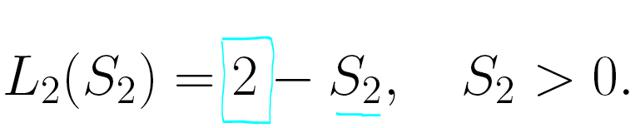(The intercept in this case "N0" = 2 and the slope "N1" = -1)

The table below represents an example originally provided by Mr. Peter Carr in his explanation of linearizing butterfly spreads. Essentially Carr's table calculates the number of butterfly spreads that are stuck at each of the remaining three possible stock prices.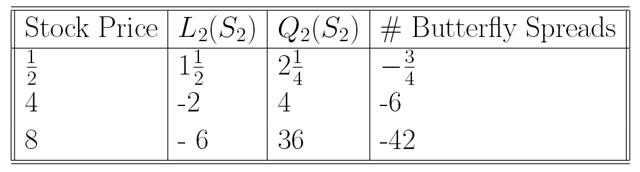The second column of the table calculates the desired linear payoff at each of the 3 remaining possible stock prices. The third column computes the quadratic payoff. Finally the fourth column calculates the number of butterfly spreads that hold at each relevant strike being the difference between the second and third columns.

The significance of Static Replication

Because curved quadratic payoff has been linearized by shorting butterfly spreads, we can finally integrate Linearity Principle. Recall that with previously mentioned zero interest rate and dividend yield, the linear payoff has zero initial value since the initial stock price is equal to 2. This factor is important and is denoted by the equation below: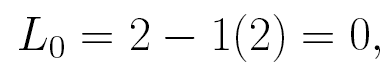In order to recover the value of the quadratic payoff, we need to consequently add back the known premiums provided by the butterfly spreads in Carr's example. In doing so, we are left with the following equation: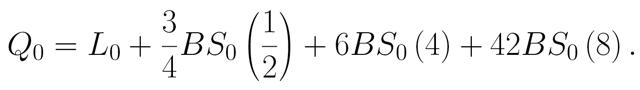In an example where we assume the 3 butterfly spreads to all cost \$ 1/9, the arbitrage-free value of the quadratic payoff would then be the following: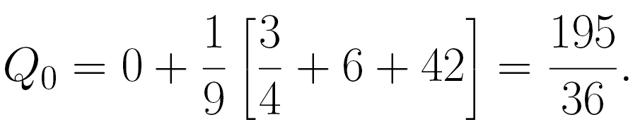Finally, this method has shown that statically it is indeed possible for the quadratic payoff to be replicated by using stock bonds and three butterfly spreads. We may also hold almost any three options in the place of the butterfly spread thus linking our observation to the trinomial model and concluding part 1 of this observation and article.

Disclosure: I/we have no positions in any stocks mentioned, and no plans to initiate any positions within the next 72 hours.

Additional disclosure: Some of the examples in this article are provided by Mr. Peter Carr in his analysis on FAQs of the binomial option pricing model in 1999. I do not proclaim ownership of those examples nor do I claim rights on the equations and theories proposed in this article. I simply wrote this article for myself and any interested readers.